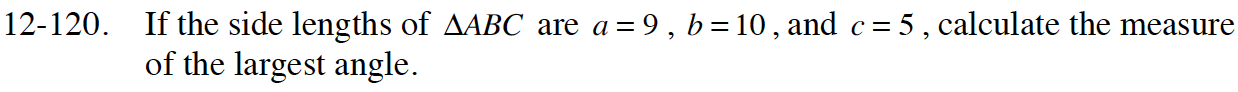Home > CCA2 > Chapter 12 > Lesson 12.2.2 > Problem12-120

12-120.

If the side lengths of ∆ABC are a = 9, b = 10, and c = 5, calculate the measure of the largest angle. Homework Help ✎Start by drawing a triangle and labeling the sides and angles.
Use the Law of Cosines, then Law of Sines to calculate the measures of the angles.
Which angle is the largest?